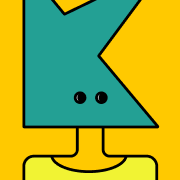# CML- Chapter 2: Sets

\$31880918404805
0 ratings
The first section in this chapter gives a historical account of set theory and why it is studied in many mathematical content courses for elementary teachers. The second part of this section considers how children develop number and then looks at the ways set theory can be used to define number and the four basic mathematical operations. The second section in this chapter describes Venn diagrams and how they may be used to organize information in mathematics as well as in other subjects.
The rationale for studying set theory is that it allows one to define the concept of number and the four basic operations associated with number (addition, subtraction, multiplication, and division). Set theory is also one of the foundations of higher mathematics.
The first section in this chapter also explores how children come to understand number. In early childhood the focus is not so much in covering
428 KB
14 pages
\$3

0 ratings